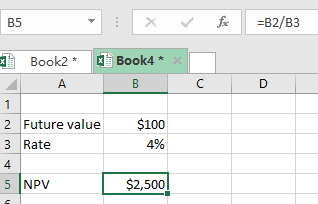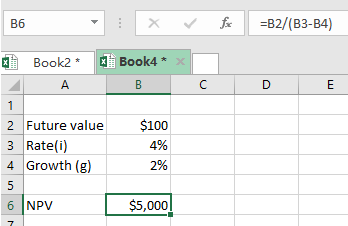Get instant live expert help with Excel or Google Sheets“My Excelchat expert helped me in less than 20 minutes, saving me what would have been 5 hours of work!”

#### Post your problem and you’ll get expert help in seconds.

Your message must be at least 40 characters
Our professional experts are available now. Your privacy is guaranteed.

# Learn How to Find the NPV of a Perpetuity in Excel

When calculating the net present value, a situation might arise where you are face with a constant series of payments without an end. This is what we refer to as NPV for a perpetuity.

To find the net present value of a perpetuity, we need to first know the future value of the investment.

## General syntax of the formula

`NPV(perpetuity)= FV/i`

Where;

• FV- is the future value
• i – is the interest rate for the perpetuity

## Example

To understand how the NPV of a perpetuity works in excel, we need to consider the example below;Figure 1: Finding NPV of perpetuity in excel

To better understand the above NPV, we need to consider a real case study;

Imagine you are evaluating a firm based on its future profits. The firm’s expected profit per year is \$100 as shown in cell B2, without an end. The cash flow is then discounted at the rate of 4% as shown in cell B3. To get the NPV, we simply divide the Future value, which is \$100, by the rate.

`=\$100/0.04`

`=\$2,500`

## What if the cash flow grows at a constant rate?

In a perpetuity case, a scenario might emerge where the cash flow increases at a given constant rate. To find the NPV in such a case, we proceed as follows;

`NPV= FV/(i-g)`

Where;

• FV– is the future value of the cash flows
• i – is the discount rate
• g- is the growth rate of the firm

## Example

Assume that a firm anticipates a profit of \$100 per year without an end. The discounted rate is 4% and the profit is expected to grow at a rate of 2% every year. What is the NPV of the perpetuity?

`NPV(perpetuity)= \$100/(0.04-0.02)`Figure 2: NPV of perpetuity with growth rate

Notice that when we have the growth rate given, the NPV is higher than that of when we don’t have a growth rate.

Most of the time, the problem you will need to solve will be more complex than a simple application of a formula or function. If you want to save hours of research and frustration, try our live Excelchat service! Our Excel Experts are available 24/7 to answer any Excel question you may have. We guarantee a connection within 30 seconds and a customized solution within 20 minutes.

Solution examplesI have a figure which is the sum of 9 months of payments. Each payment decreases by the same amount (X) so the first payment is Y, second payment is Y-X, third is Y-(Xx2), forth is Y-(Xx3) and so on. I am trying to calculate what each of the 9 payments should be.
Solved by A. W. in 60 minshow do I calculate daily interest in excel? for instance if I have \$20,000 in a bank that gives .5% interest and calculates it daily, I want to create a spreadsheet that will show what I will have at the end of a 30 day month
Solved by O. A. in 21 minshow would i do this question on excel? An investment will generate Â£15,000 a year for 20 years. If you require a rate of compensation of 10% and the investment costs Â£100,000, show whether it is worth buying? If expected inflation is instead estimated to be 2% higher, show how this impacts on your recommendation.
Solved by V. W. in 60 mins